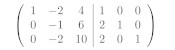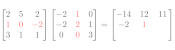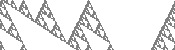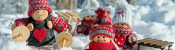mscroggs.co.uk
mscroggs.co.uksubscribe

# Blog

## Inverting a matrix

2020-02-06
 This is the third post in a series of posts about matrix methods.
Yet again, we want to solve $$\mathbf{A}\mathbf{x}=\mathbf{b}$$, where $$\mathbf{A}$$ is a (known) matrix, $$\mathbf{b}$$ is a (known) vector, and $$\mathbf{x}$$ is an unknown vector.
In the previous post in this series, we used Gaussian elimination to invert a matrix. You may, however, have been taught an alternative method for calculating the inverse of a matrix. This method has four steps:
1. Find the determinants of smaller blocks of the matrix to find the "matrix of minors".
2. Multiply some of the entries by -1 to get the "matrix of cofactors".
3. Transpose the matrix.
4. Divide by the determinant of the matrix you started with.

### An example

As an example, we will find the inverse of the following matrix.
$$\begin{pmatrix} 1&-2&4\\ -2&3&-2\\ -2&2&2 \end{pmatrix}.$$
The result of the four steps above is the calculation
$$\frac1{\det\begin{pmatrix} 1&-2&4\\ -2&3&-2\\ -2&2&2 \end{pmatrix} }\begin{pmatrix} \det\begin{pmatrix}3&-2\\2&2\end{pmatrix}& -\det\begin{pmatrix}-2&4\\2&2\end{pmatrix}& \det\begin{pmatrix}-2&4\\3&-2\end{pmatrix}\\ -\det\begin{pmatrix}-2&-2\\-2&2\end{pmatrix}& \det\begin{pmatrix}1&4\\-2&2\end{pmatrix}& -\det\begin{pmatrix}1&4\\-2&-2\end{pmatrix}\\ \det\begin{pmatrix}-2&3\\-2&2\end{pmatrix}& -\det\begin{pmatrix}1&-2\\-2&2\end{pmatrix}& \det\begin{pmatrix}1&-2\\-2&3\end{pmatrix} \end{pmatrix}.$$
Calculating the determinants gives $$\frac12 \begin{pmatrix} 10&12&-8\\ 8&10&-6\\ 2&2&-1 \end{pmatrix},$$ which simplifies to
$$\begin{pmatrix} 5&6&-4\\ 4&5&-3\\ 1&1&-\tfrac12 \end{pmatrix}.$$

### How many operations

This method can be used to find the inverse of a matrix of any size. Using this method on an $$n\times n$$ matrix will require:
1. Finding the determinant of $$n^2$$ different $$(n-1)\times(n-1)$$ matrices.
2. Multiplying $$\left\lfloor\tfrac{n}2\right\rfloor$$ of these matrices by -1.
3. Calculating the determinant of a $$n\times n$$ matrix.
4. Dividing $$n^2$$ numbers by this determinant.
If $$d_n$$ is the number of operations needed to find the determinant of an $$n\times n$$ matrix, the total number of operations for this method is
$$n^2d_{n-1} + \left\lfloor\tfrac{n}2\right\rfloor + d_n + n^2.$$

### How many operations to find a determinant

If you work through the usual method of calculating the determinant by calculating determinants of smaller blocks the combining them, you can work out that the number of operations needed to calculate a determinant in this way is $$\mathcal{O}(n!)$$. For large values of $$n$$, this is significantly larger than any power of $$n$$.
There are other methods of calculating determinants: the fastest of these is $$\mathcal{O}(n^{2.373})$$. For large $$n$$, this is significantly smaller than $$\mathcal{O}(n!)$$.

### How many operations

Even if the quick $$\mathcal{O}(n^{2.373})$$ method for calculating determinants is used, the number of operations required to invert a matrix will be of the order of
$$n^2(n-1)^{2.373} + \left\lfloor\tfrac{n}2\right\rfloor + n^{2.373} + n^2.$$
This is $$\mathcal{O}(n^{4.373})$$, and so for large matrices this will be slower than Gaussian elimination, which was $$\mathcal{O}(n^3)$$.
In fact, this method could only be faster than Gaussian elimination if you discovered a method of finding a determinant faster than $$\mathcal{O}(n)$$. This seems highly unlikely to be possible, as an $$n\times n$$ matrix has $$n^2$$ entries and we should expect to operate on each of these at least once.
So, for large matrices, Gaussian elimination looks like it will always be faster, so you can safely forget this four-step method.
Previous post in seriesGaussian elimination
This is the third post in a series of posts about matrix methods.

### Similar postsGaussian eliminationMatrix multiplicationClose encounters of the second kindChristmas (2020) is over

Comments in green were written by me. Comments in blue were not written by me.